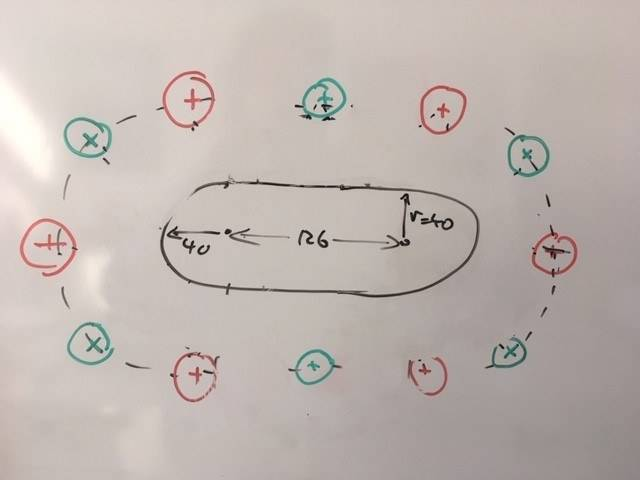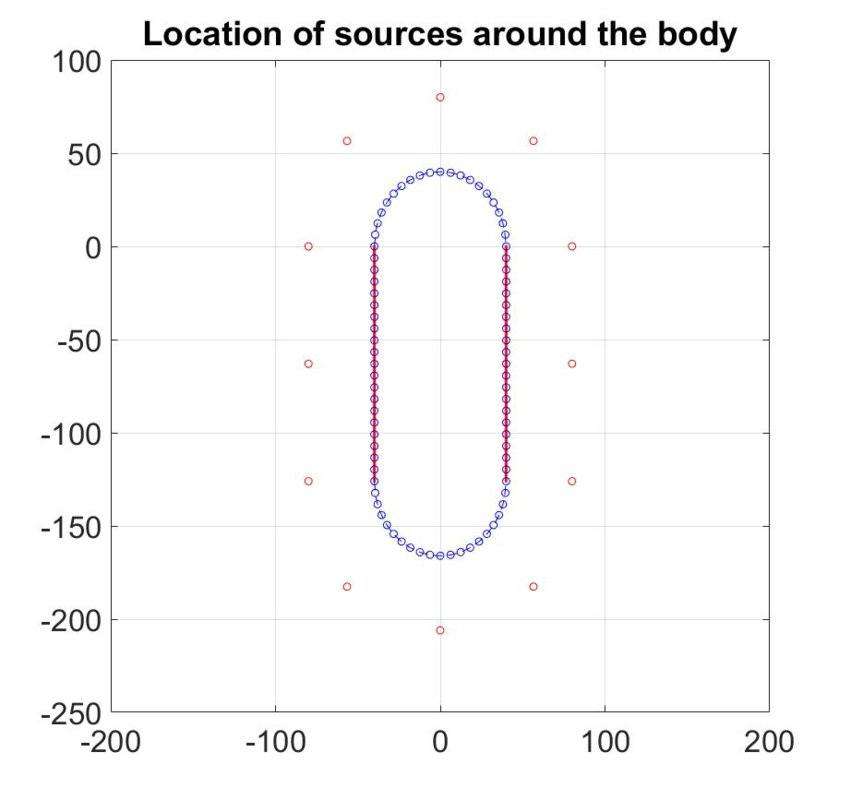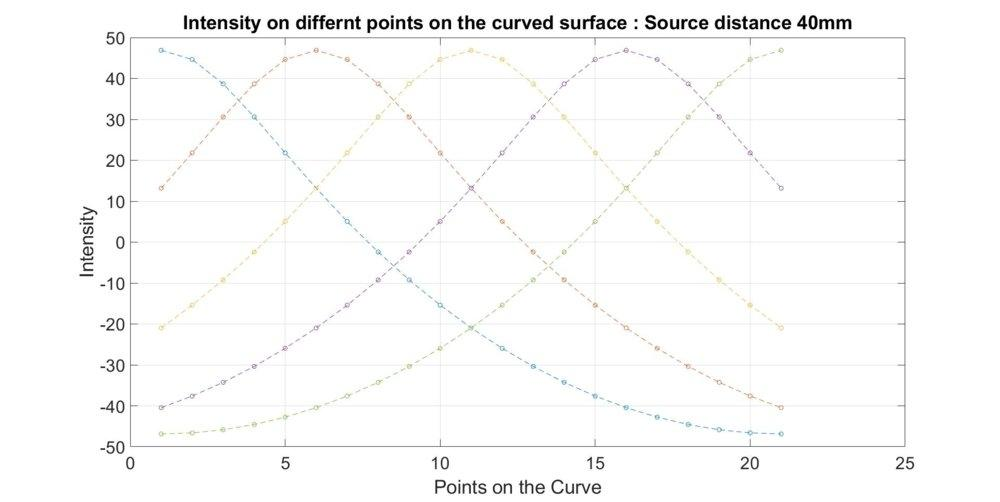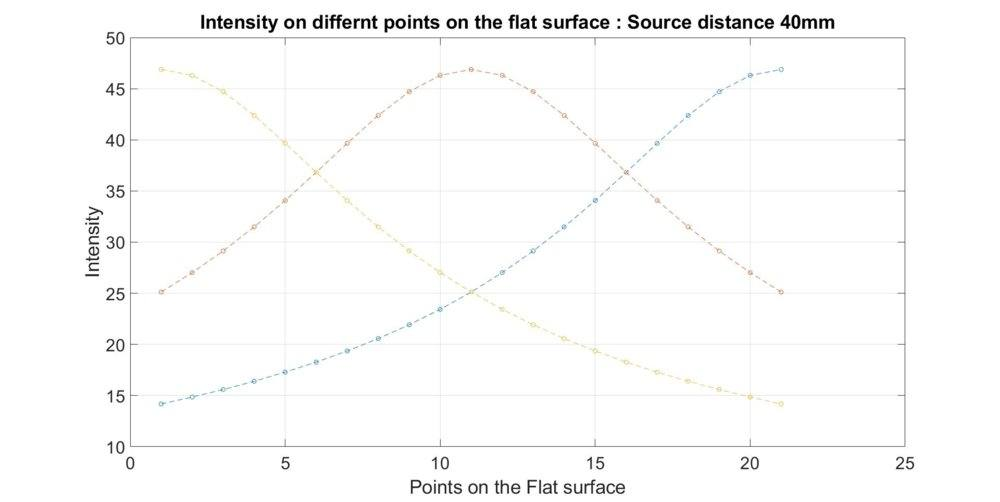# Radiation heat transfer using 12 heat sources

• imselva
In summary, the intensity at a point on a flat surface is proportional to the square of the distance from the source and depends on the angle of incidence.

#### imselva

TL;DR Summary
I want to have a constant distribution of heat flux around a muffle chamber (shown in fig) using 12 Radiation heat sources.
The distance bewteen the Body wall and the sources should be in between 40 and 80mm. The distance between the sources should also be kept constant.The intensity is proportional to the square of the distance between the source and the body and also depends on the angle of incident.
I = The intensity at 0° (Assuming I = some constant)
then I0 = I cos(Theta)
Theta = the angle of incidence.
I want to solve this using trignomentry. Is it possible?
How shall I proceed with this Problem. I want to have the same value of I all over the body considered.
This is a problem scratching my head for over a week now.

Yes, you can do this with trigonometry and the Stefan-Boltzmann equation. You need all of the geometric details of the setup and the temperature and emissivity of the emitters and emissivity of the "muffle chamber" (furnace?). The heat transfer depends on the temperatures of the surfaces and the angle subtended. And remember; any angle not covered by emitter is emission by the target against the surroundings.

Also, you will need to tell us what problem you are trying to solve; all you said is you want to "solve this". Solve what? I'll take a guess that you are interested in the steady state temperature of the chamber...

•imselva and Lnewqban
Sorry that I did not mention that.
I am trying to move the heating Elements around the "Muffle chamber"(Yes it is a furnace in a Simulator used to heat the specimen) so that I get the same intensity of Radiation at all Points on the muffle chamber.

GOAL : To achieve same intensity on all Points on the Surface of the Muffle chamber.

Initially I tried to keep the heating Elements as shown in figure and calculated the intensity of the Radiation on the Surface (on a certain number of points). But there's always some deviation (When plotted, the intensity on the Surface is not a straight line).
So i came to the conclusion that keeping the heating Elements in the axis as shown in the figure won't give the required result.

The usual approach is to wrap a resistance heating element around the furnace. That works for cylindrical work areas where the important part is in the middle section.

For other shapes, you probably need to apply the heater in a serpentine pattern, which of course can vary depending on the local shape.

Don't forget to use LOTS of thermal insulation if you need very even temperatures throughout the work volume. You may also need separate temperature control zones at the extremeties.

Cheers,
Tom

•imselva
Yes that is the usual approach. But due to some constraints I have to stick with this.

The intensity of the heat flux from the Sources varies with the angle of incidence.

To my understanding and knowledge if a Point on the work volume if getting heat flux from 2 sources, say A and B. Say the heat flux from A is greater than the heat flux from B. The intensity of the heat flux at the Point under consideration will be equal to the intensity of the heat flux from A.

I think the heat flux intensities won't add up. Am I correct?

If i am correct, I think it is impossible to obtain uniform heat intensities at all the Points in the Surface.

If acheiving uniform heat intensities on all Points on the Surface is possible, please let me know how to achieve it.

Thank you

I believe you have over-constrained the solution.
The distance between radiators and from the muffle needs to be varied to get the best even illumination. Are the heat sources fixed-width area radiators or are they separated line radiators? Is there a reflector outside the radiators? Are the radiators all operated at the same temperature?

Can we assume that non-coherent energy is incident on the muffle surface, and that energy sums. Hotter areas of the muffle will reflect more energy.

The heat Sources can be moved (Maximum distance between the wall and the heat source is 80mm).
I ran the calculations in MATLAB by varaying the distance between the Radiators and the muffle.

The heat source is a cylinder of 19mm Diameter. The whole set up is completely insulated and there is very Minimum loss to the surroundings. All the heat Radiators are operated at the same temperature for now.

Can the intensity from two different non-coherent Sources on a Point on the surface be summed together?

Since you need radiant heating you are in the realm of optics. You may recall that light intensity falls of with the square of distance from the source.

Using a flat work surface as an example with a point source, the flux falloff will be proportional to cos2α, where α is the angle measure between the ray from the source, and the line that runs normal to the surface to the source. Hmm... this doesn't explain well with words!

Take a flashlight and aim it perpendicular to a wall. α is the angle from the center of the flashlight lens to the edge of the spot on the wall.

This demonstrates that you will need some optics to beamshape the source to get even work illumination. The most obvious approach would be a reflector at the source to attempt a linear falloff with angle, rather than a cos2 falloff. A modification of a parabolic reflector comes to mind, but the math is beyond me.

An additional factor is the shape of the work piece. If it is 3-dimensional you also have the falloff where there is not surface-normal to the source.

This paper might be of some use:
https://nvlpubs.nist.gov/nistpubs/jres/39/jresv39n3p213_A1b.pdf
(this, and others found with:

edit: Since you have external insulation, you could try heating the insulation surface relatively evenly while blocking direct heater radiation to the work.

Cheers,
Tom

•imselvaThis is how I stared the Problem. I took the flat Surface on the left side and placed 20 Points on ist Surface. Also I took the top curved Surface and placed 20 Points on it.
I calculated the angle of incidence for all the considered Points on the Surface.

I already took into account the inverse square law and lambert's cosine law.
I = I' Cos(Theta).
I' = Intensity at Zero Degree / (distance between source and the wall)^2)

And I got the following resultIgnore the values on the negative side it is irrelevant.As you can see the Variation of Intensities on the Point considered.
I want to have uniform heat intensities on all Points considered.

Can we add the intensities from two different Sources?
Say for example I think the intensity on Point 1 will be 46 and not (46+25+12) 25 and 12 are the contribution from other Sources.

Am I correct?

Thank you.

Yes, they will add... just like two lightbulbs in a lamp, the place get darker when one bulb fails. That was taken advantage of in the incandescent 3-way lightbulbs, they had two filaments and the 3-way was one filament, the other, both.

•imselva
So can I proceed by adding the intensity contribution from multiple sources?
In the example I quoted in the previous Reply, the intensity at Point 1 is the sum of 46,25 and 12 i.e. 83. Not just 46.
Is there any kind of basic physics behind this addition of intensity. So that I could study more about this and understand it completely.

Thank you very much

imselva said:
Is there any kind of basic physics behind this addition of intensity.
"...cannot be created or destroyed..."A real-world parallel could be sifting sand. You manually shovel some sand onto a screen to remove rocks from it. Now someone comes over, picks up a shovel, and shovels it onto the screen.

Or more directly, back to turning on that second lamp in the room; or shining two flashlight at the same spot.

•imselva
The fundamental problem here is that you are trying to evenly illuminate a convex surface from a point source. The inverse square law and the curve of the convex surface make that impossible. It will require a curved mirror or a different shaped muffle.

If there will be a thermal equilibrium over some time, with energy traveling in both directions, you might replace the thin muffle with a thicker copper muffle that is able to transport heat around the walls. If you were exposing a curved photograph with a single flash the problem would be different.

Similar geometrical radiative problems will occur between the contents of the muffle and the muffle itself. What is being heated? How long will it be allowed to achieve thermal stability?

•imselva, Tom.G and russ_watters